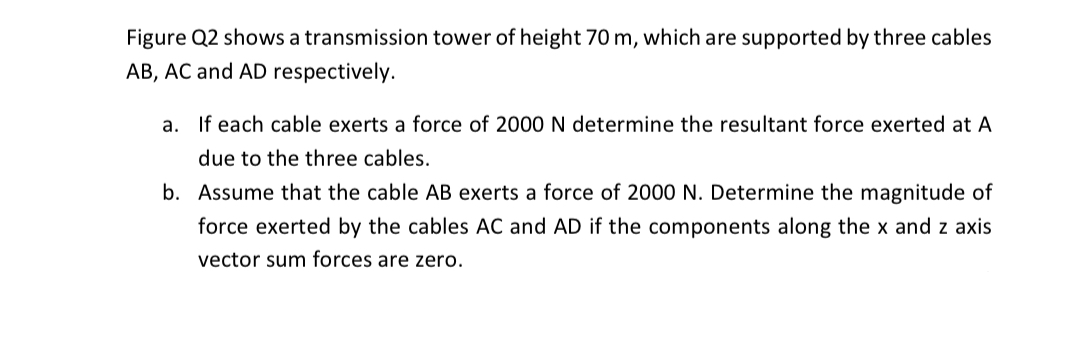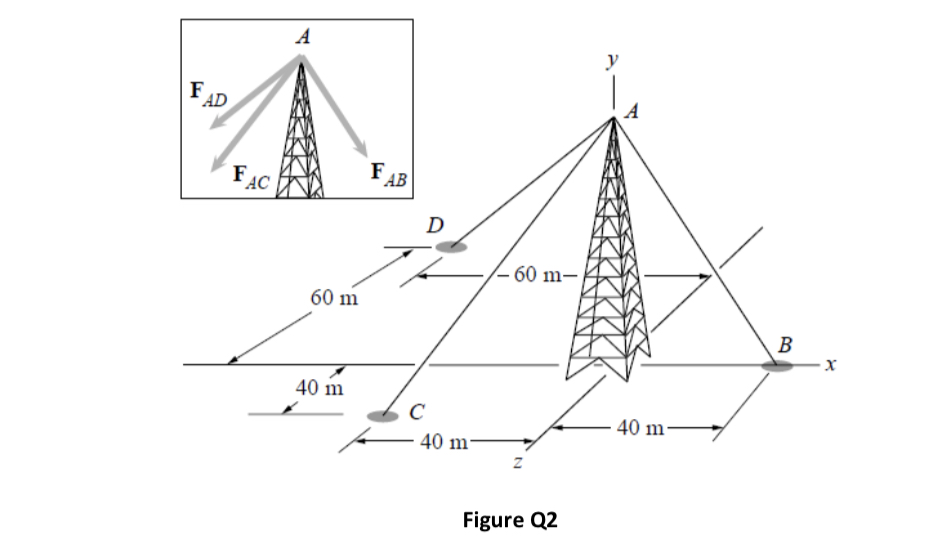# Figure Q2 shows a transmission tower of height 70 m, which are supported by three cables AB, AC and AD respectively. a. If each cable exerts a force of 2000 N determine the resultant force exerted at A due to the three cables. b. Assume that the cable AB exerts a force of 2000 N. Determine the magnitude of force exerted by the cables AC and AD if the components along the x and z axis vector sum forces are zero.

Questionhelp_outlineImage TranscriptioncloseFigure Q2 shows a transmission tower of height 70 m, which are supported by three cables AB, AC and AD respectively. a. If each cable exerts a force of 2000 N determine the resultant force exerted at A due to the three cables. b. Assume that the cable AB exerts a force of 2000 N. Determine the magnitude of force exerted by the cables AC and AD if the components along the x and z axis vector sum forces are zero. fullscreenfullscreen

### Want to see this answer and more?

Experts are waiting 24/7 to provide step-by-step solutions in as fast as 30 minutes!*

*Response times may vary by subject and question complexity. Median response time is 34 minutes for paid subscribers and may be longer for promotional offers.
Tagged in
Engineering
Mechanical Engineering

### Classical Mechanics